Latest Banking jobs   »

# Quantitative Aptitude Quiz For Bank Foundation 2023 14th January

Directions (1-5): What will come in place of question mark (?) in the following questions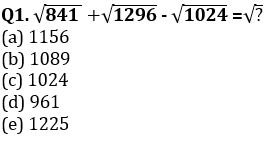Q2. 14400÷36+15600 ÷12 + 450 = 1800+ ?
(a) 410
(b) 330
(c) 390
(d) 350
(e) 370

Q3. 7450 +5880 – 6890 = 9000 – ?
(a) 2560
(b) 2760
(c) 2460
(d) 2850
(e) 2480

Q4. 32×25+44×18+348÷6 = ?
(a) 1550
(b) 1620
(c) 1650
(d) 1600
(e) 1690

Q5.√1225×28+203×7 = (?)²
(a)47
(b)45
(c) 49
(d) 51
(e) 53

Q6. The average expenditure of Nandu & Bandu on rent is Rs. 2000 while that on travel is Rs. 1500. Nandu spends Rs. 800 on food while Bandu spends Rs. 900. What is average of total expenditure of both? (no one spends on any other thing than given)
(a) 4400
(b) 4350
(c) 4300
(d) 4750
(e) 4800

Q7. Anu bought a purse at a discount of 20% which was marked at 30% higher than cost price. A customer gets 10% extra discount on purchase of more than two purse. If Anu purchased 3 such purses, find profit or loss percent of shopkeeper.
(a) 4% loss
(b) 4% profit
(c) 6.4% profit
(d) 6.4% loss
(e) None of these

Q8. What is the probability of drawing 2 red cards from a pack of 52 cards having 2 black cards missing?
(a) 13/49
(b) 25/153
(c) 25/102
(d) 69/221
(e) 13/25

Q9. In a bag there are 2 red balls, X green balls and 3 yellow balls. If two balls are taken out, then its probability to be green is 2/9. Find number of green balls in the bag.
(a) 8
(b) 4
(c) 5
(d) 6
(e) 7

Q10. Kappu & Chandu have their speed in ratio 5:6. If both start from 2 points 110 kms away towards each other. How much distance Chandu had travelled more than Kappu when they meet for first time? (both start at same time)
(a) 11 kms
(b) 20 kms
(c) 10 kms
(d) Cannot be determined
(e) None of these

Direction(11-15): In each of these questions, two equations (I) and (II) are given. Solve the equations and mark the correct option:

Q11.
I: 2x² + 10x + 12 = 0
II: y² + 10x + 25 = 0
(a) x > y
(b) x ≥ y
(c) x < y
(d) x ≤ y
(e) x = y or relation can’t be established.

Q12.
I. x² – 5x + 6 = 0
II. y² + 7y + 6 = 0
(a) x > y
(b) x ≥ y
(c) x < y
(d) x ≤ y
(e) x = y or relation can’t be established.

Q13.
I. x² = 625
II. y = √625
(a) x > y
(b) x ≥ y
(c) x < y
(d) x ≤ y
(e) x = y or relation can’t be established.

Q14.
I. 2x – 3y = 0
II. 4x – 2y = 16
(a) x > y
(b) x ≥ y
(c) x < y
(d) x ≤ y
(e) x = y or relation can’t be established.

Q15.
I. x³ = 1331
II. y = ∛1331
(a) x > y
(b) x ≥ y
(c) x < y
(d) x ≤ y
(e) x = y or relation can’t be established.

Solutions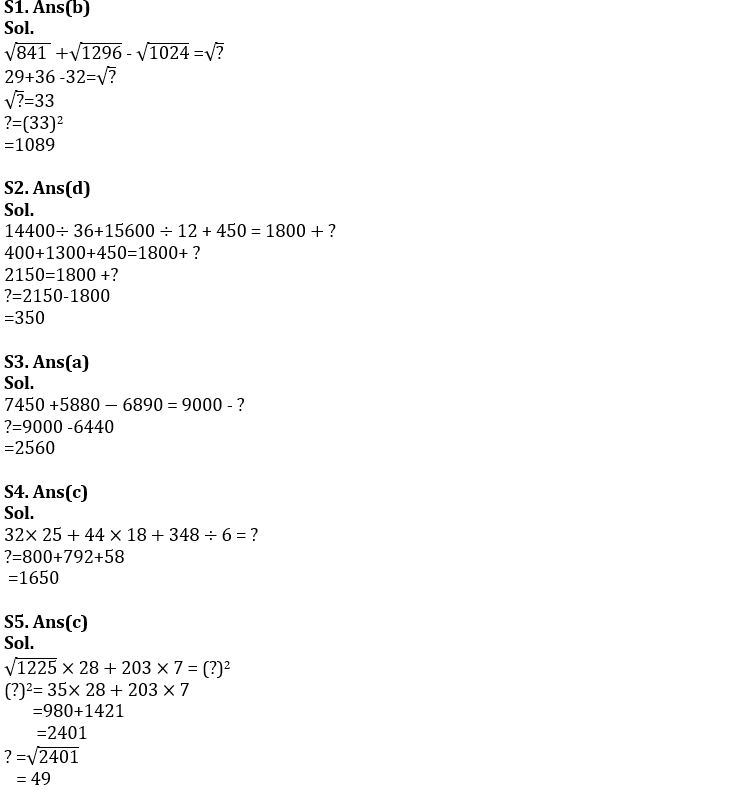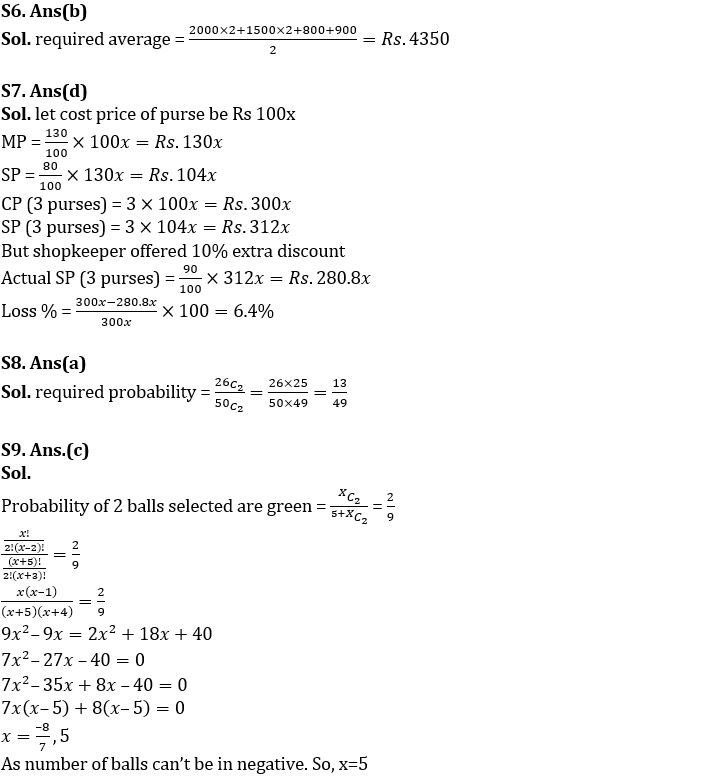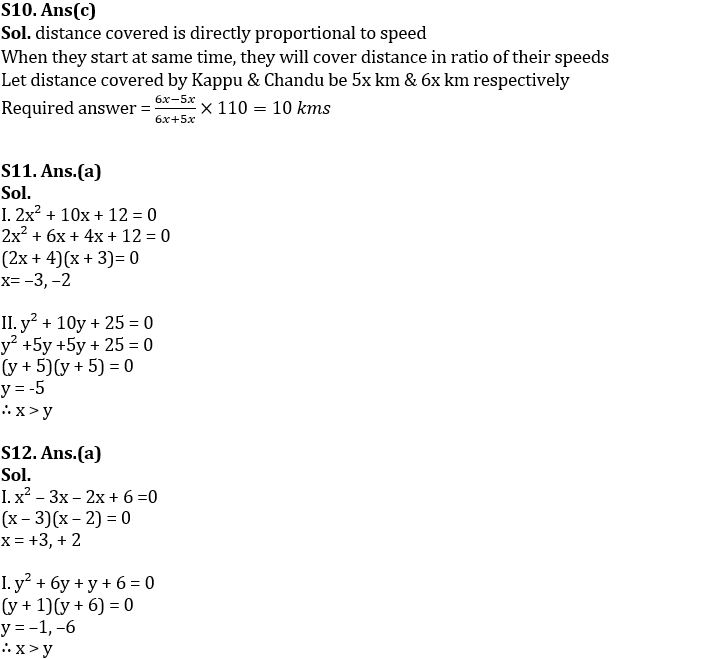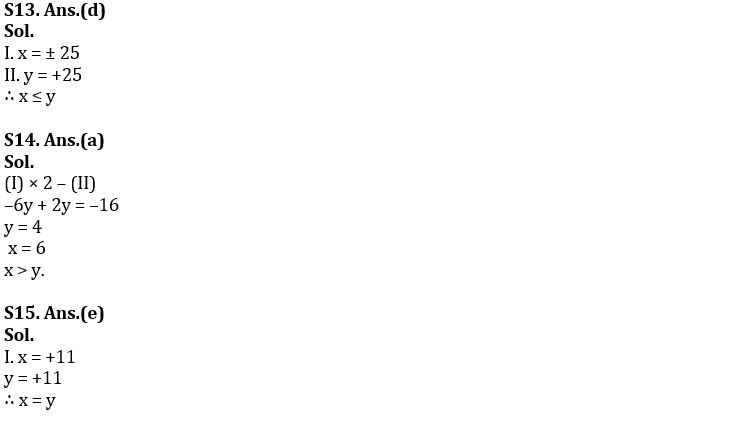## FAQs

### What is the selection process of the Bank Clerk?

The selection process of the Bank Clerk is Prelims & Mains.

#### Congratulations!